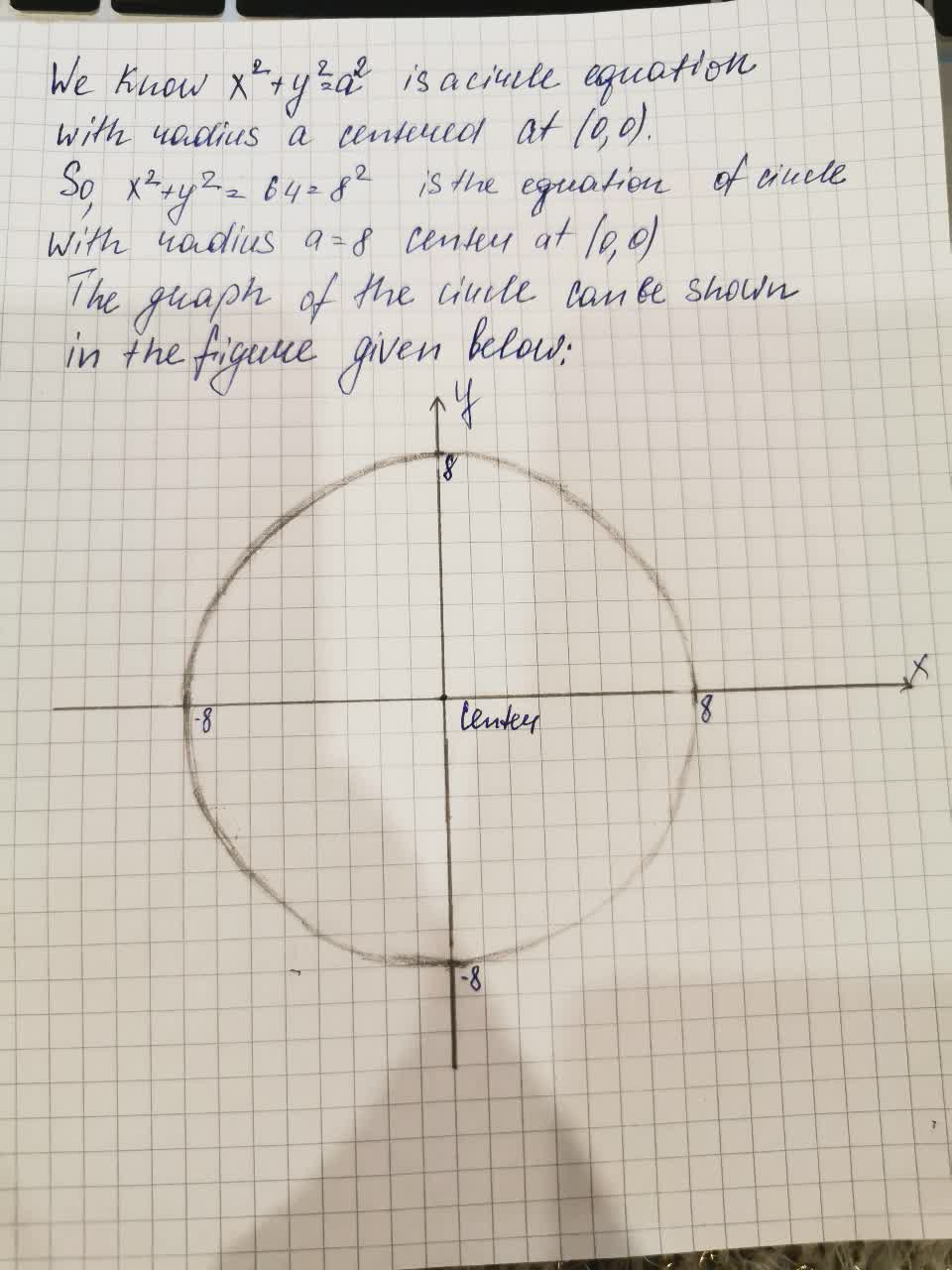# Is it true that the equations r=8, x^2+y^2=64, and x=8sin(3t), y=8cos(3t) (0<=t<=2pi) all have the same graph.aflacatn 2021-08-16 Answered
Is it true that the equations r=8, $$\displaystyle{x}^{{2}}+{y}^{{2}}={64}$$, and $$\displaystyle{x}={8}{\sin{{\left({3}{t}\right)}}},{y}={8}{\cos{{\left({3}{t}\right)}}}{\left({0}\le{t}\le{2}\pi\right)}$$ all have the same graph.

• Questions are typically answered in as fast as 30 minutes

### Plainmath recommends

• Get a detailed answer even on the hardest topics.
• Ask an expert for a step-by-step guidance to learn to do it yourself.Aubree Mcintyre2.Now, we will check that what type of equation is the r=8
This equation is the polar form equation in which we consider $$\displaystyle{r}=\sqrt{{{x}^{{2}}+{y}^{{2}}}}$$
So, $$\displaystyle{r}=\sqrt{{{x}^{{2}}+{y}^{{2}}}}={8}$$
$$\displaystyle\Rightarrow={x}^{{2}}+{y}^{{2}}={8}^{{2}}={64}$$
Thus, we can say that after changing polar co-ordinates to Cartesian co-ordinates, equation  r=8  is also the equation of the circle with radius a=8 and center at (0,0).
So, the graphs of the equation
r=8 and $$\displaystyle{x}^{{2}}+{y}^{{2}}={64}$$ are same which is given above.
Now, we have the parametric form equations given as:
$$\displaystyle{x}={8}{\sin{{\left({3}{t}\right)}}},{y}={8}{\cos{{\left({3}{t}\right)}}},{0}\le{t}\le{2}\pi$$
$$\displaystyle{x}^{{2}}={64}{{\sin}^{{2}}{\left({3}{t}\right)}}$$
and $$\displaystyle{y}^{{2}}={64}{{\cos}^{{2}}{\left({3}{t}\right)}}$$
$$\displaystyle\Rightarrow{x}^{{2}}+{y}^{{2}}={64}{{\sin}^{{2}}{\left({3}{t}\right)}}+{64}{{\cos}^{{2}}{\left({3}{t}\right)}}$$
$$\displaystyle={64}{\left({{\sin}^{{2}}{\left({3}{t}\right)}}+{{\cos}^{{2}}{\left({3}{t}\right)}}\right)}={64}$$
as by trigonometry identity $$\displaystyle{{\sin}^{{2}}{\left({3}{t}{0}+{{\cos}^{{2}}{\left({3}{t}\right)}}={1}\right.}}$$
thus, we have shown that these parametric equations from circle with radius 8 and center at (0,0).
So, these parametric equations
$$\displaystyle{x}={8}{\sin{{\left({3}{t}\right)}}},{y}={8}{\cos{{\left({t}\right)}}},{0}\le{t}\le{2}\pi$$ also have the same graph given above.
4. So, the given statement is true as the equation
r=8 ,
$$\displaystyle{x}^{{2}}+{y}^{{2}}={64}$$ and
$$\displaystyle{x}={8}{\sin{{\left({3}{t}\right)}}},{y}={8}{\cos{{\left({t}\right)}}},{0}\le{t}\le{2}\pi$$ denote same equation of circle with radius 8 and center at
origin.Thus, all these equations have the same graph given above.